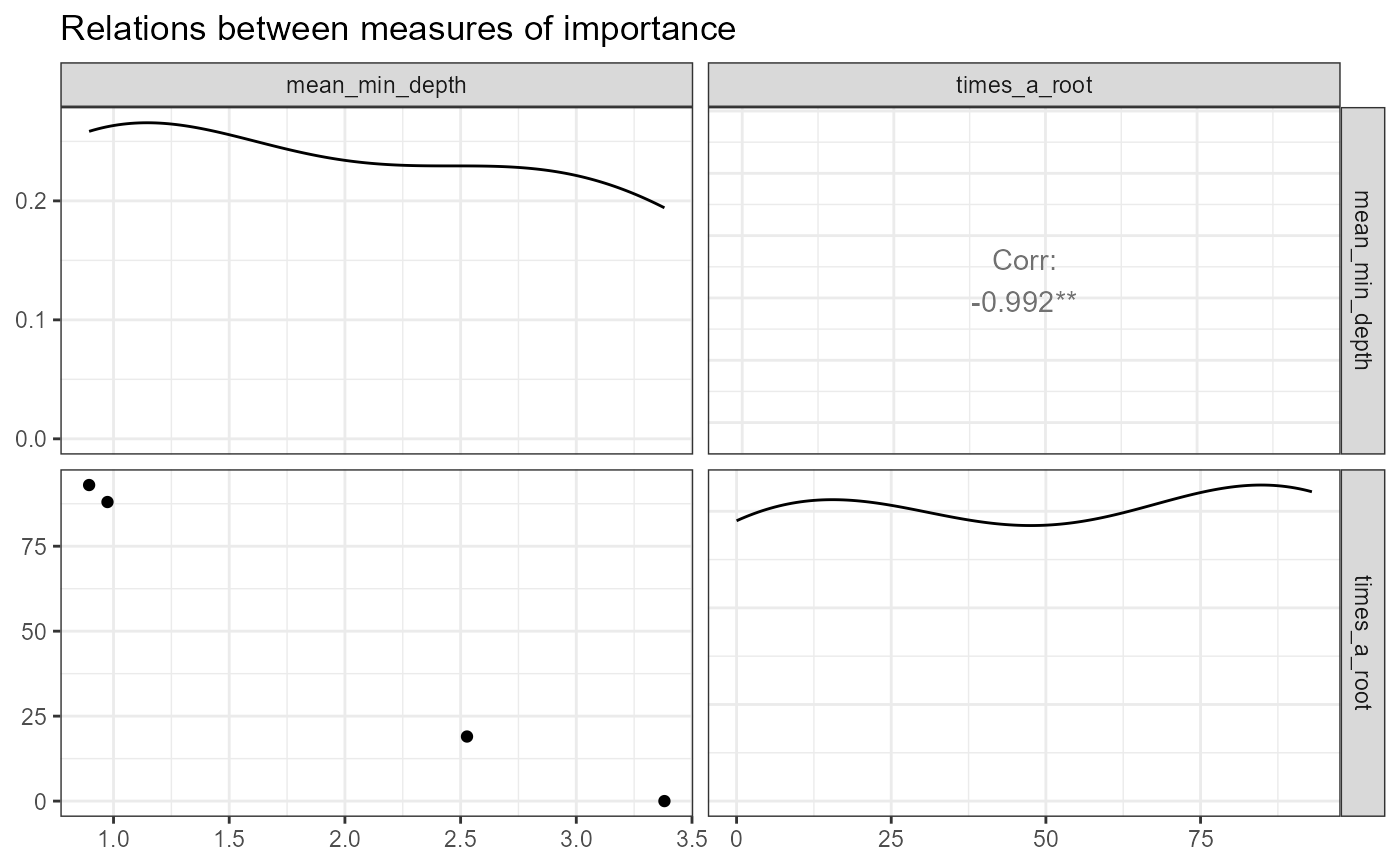Plot selected measures of importance of variables in a forest using ggpairs

plot_importance_ggpairs(
importance_frame,
measures = NULL,
main = "Relations between measures of importance"
)

## Arguments

importance_frame A result of using the function measure_importance() to a random forest or a randomForest object A character vector specifying the measures of importance to be used A string to be used as title of the plot

A ggplot object

## Examples

forest <- randomForest::randomForest(Species ~ ., data = iris, localImp = TRUE, ntree = 200)
frame <- measure_importance(forest, measures = c("mean_min_depth", "times_a_root"))
plot_importance_ggpairs(frame, measures = c("mean_min_depth", "times_a_root"))